• ## Resnet

2021-03-14 17:02:42
原文地址：https://www.jianshu.com/p/93990a641066 介绍 终于可以说一下Resnet分类网络了，它差不多是当前应用最为广泛的CNN特征提取网络。它的提出始于2015年，作者中间有大名鼎鼎的三位人物He-Kaiming, Ren-...
Resnet
原文地址：https://www.jianshu.com/p/93990a641066
介绍
终于可以说一下Resnet分类网络了，它差不多是当前应用最为广泛的CNN特征提取网络。它的提出始于2015年，作者中间有大名鼎鼎的三位人物He-Kaiming, Ren-Shaoqing, Sun-Jian。绝对是华人学者的骄傲啊。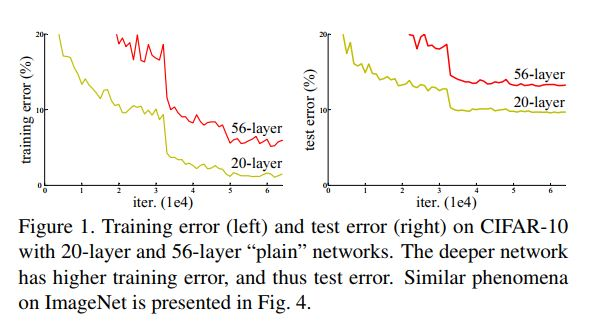常规CNN网络后期层数增加带来的分类准确率的降低
正是受制于此不清不楚的问题，VGG网络达到19层后再增加层数就开始导致分类性能的下降。而Resnet网络作者则想到了常规计算机视觉领域常用的residual representation的概念，并进一步将它应用在了CNN模型的构建当中，于是就有了基本的residual learning的block。它通过使用多个有参层来学习输入输出之间的残差表示，而非像一般CNN网络（如Alexnet/VGG等）那样使用有参层来直接尝试学习输入、输出之间的映射。实验表明使用一般意义上的有参层来直接学习残差比直接学习输入、输出间映射要容易得多（收敛速度更快），也有效得多（可通过使用更多的层来达到更高的分类精度）。
深度残差学习（Deep Residual learning）
残差学习
若将输入设为X，将某一有参网络层设为H，那么以X为输入的此层的输出将为H(X)。一般的CNN网络如Alexnet/VGG等会直接通过训练学习出参数函数H的表达，从而直接学习X -> H(X)。
而残差学习则是致力于使用多个有参网络层来学习输入、输出之间的参差即H(X) - X即学习X -> (H(X) - X) + X。其中X这一部分为直接的identity mapping，而H(X) - X则为有参网络层要学习的输入输出间残差。
下图为残差学习这一思想的基本表示。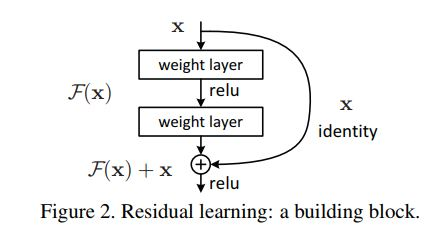残差学习的基本单元
Identity mapping
上小节中，我们知道残差学习单元通过Identity mapping的引入在输入、输出之间建立了一条直接的关联通道，从而使得强大的有参层集中精力学习输入、输出之间的残差。一般我们用F(X, Wi)来表示残差映射，那么输出即为：Y = F(X, Wi) + X。当输入、输出通道数相同时，我们自然可以如此直接使用X进行相加。而当它们之间的通道数目不同时，我们就需要考虑建立一种有效的identity mapping函数从而可以使得处理后的输入X与输出Y的通道数目相同即Y = F(X, Wi) + Ws*X。
当X与Y通道数目不同时，作者尝试了两种identity mapping的方式。一种即简单地将X相对Y缺失的通道直接补零从而使其能够相对齐的方式，另一种则是通过使用1x1的conv来表示Ws映射从而使得最终输入与输出的通道达到一致的方式。
实验比较所用到的残差网络结构与朴素网络结构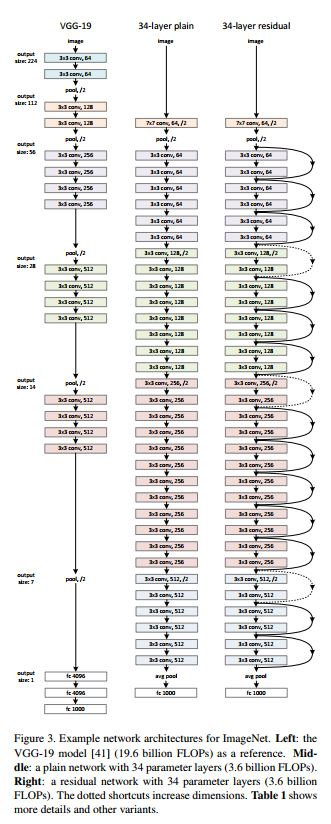残差网络与朴素网络结构之间的对比
作者为了表明残差网络的有效性，共使用了三种网络进行实验。其一为VGG19网络（这是VGG paper中最深的亦是最有效的一种网络结构），另外则是顺着VGG网络思维继续加深其层次而形成的一种VGG朴素网络，它共有34个含参层。最后则是与上述34层朴素网络相对应的Resnet网络，它主要由上节中所介绍的残差单元来构成。
在具体实现残差网络时，对于其中的输入、输出通道数目不同的情况作者使用了两种可能的选择。A)shortcut直接使用identity mapping，不足的通道通同补零来对齐；B)使用1x1的Conv来表示Ws映射，从而使得输入、输出通道数目相同。
自下面两表中，我们可看出残差网络能够在深度增加的情况下维持强劲的准确率增长，有效地避免了VGG网络中层数增加到一定程度，模型准确度不升反降的问题。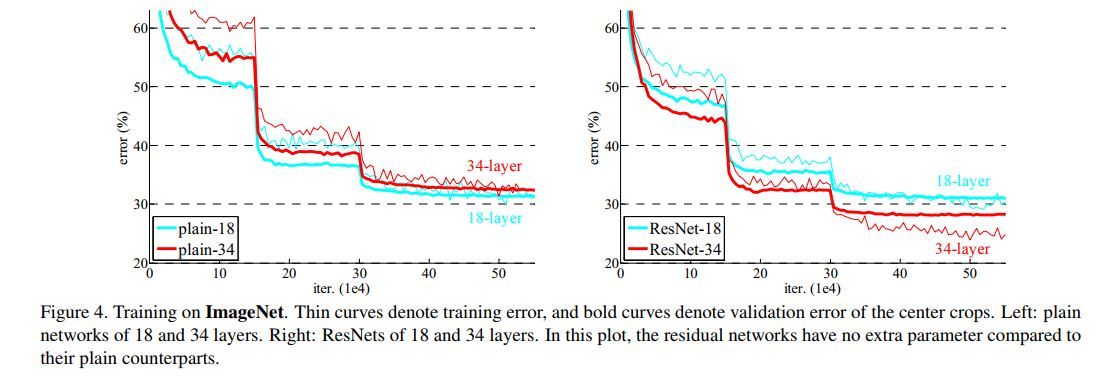不同深度的朴素网络与残差网络在Imagenet上的性能表现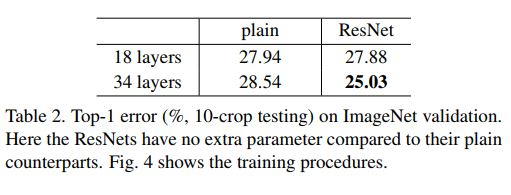不同深度的朴素网络与残差网络在Imagenet上的性能表现其二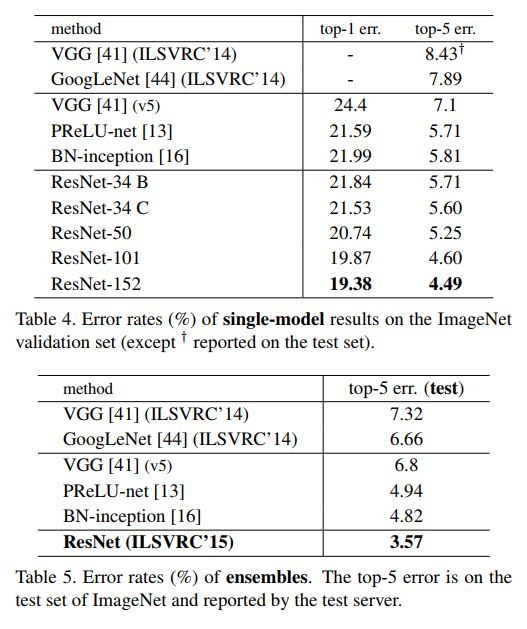Resnet常规网络与其它模型之间的性能比较
bottleneck构建模块
为了实际计算的考虑，作者提出了一种bottleneck的结构块来代替常规的Resedual block，它像Inception网络那样通过使用1x1 conv来巧妙地缩减或扩张feature map维度从而使得我们的3x3 conv的filters数目不受外界即上一层输入的影响，自然它的输出也不会影响到下一层module。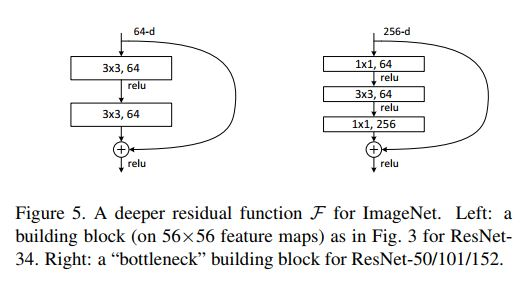Bottleneck模块
不过它纯是为了节省计算时间进而缩小整个模型训练所需的时间而设计的，对最终的模型精度并无影响。
CIFAR10上更深的Resnet网络
作者进一步在小的CIFAR10数据集上尝试了更深的Resnet网络，其深度最多达到了1202层。不过却发现分类性能终于开始有了一定下降。作者分析认为可能是层数过多，导致模型过于复杂，而CIFAR-10较小的数据集造成了它的过拟合吧。
如下表为其疯狂的实验结果。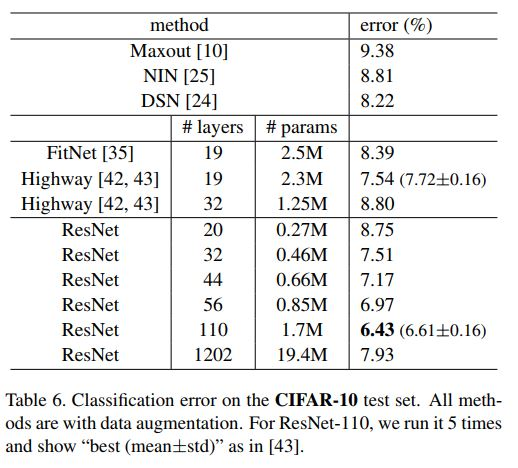较小的数据集造成了它的过拟合吧。
如下表为其疯狂的实验结果。
[外链图片转存中…(img-yizg6EwK-1615712532858)]
CIFAR-10上更深的Resnet网络的应用


展开全文人工智能 深度学习 计算机视觉
• ## ResNet

2018-12-05 21:06:15
原文地址： Deep Residual Learning for Image Recognition 作者：Kaiming He, Xiangyu Zhang, Shaoqing Ren, Jian Sun 成就：模型赢得了ImageNet检测任务、ImageNet定位任务、COCO检测和COCO分割任务的第一名。 ...
文章目录论文信息ResNet 设计动机深度残差学习（deep residual learning）恒等映射块结构ResNet 网络架构ResNet 50 微调
论文信息
原文地址： Deep Residual Learning for Image Recognition
作者：Kaiming He, Xiangyu Zhang, Shaoqing Ren, Jian Sun
成就：模型在ImageNet ILSVRC和COCO 2015上，一共取得了5项冠军。
ResNet 设计动机
在深度卷积神经网络中，增加网络层数意味着网络可以进行更加复杂的特征模式的提取。理论上，更深的模型可以取得更好的结果，对于梯度消失/爆炸的问题，也可以通过标准初始化方法（normalized initialization）和归一化层（normalization layers）进行解决。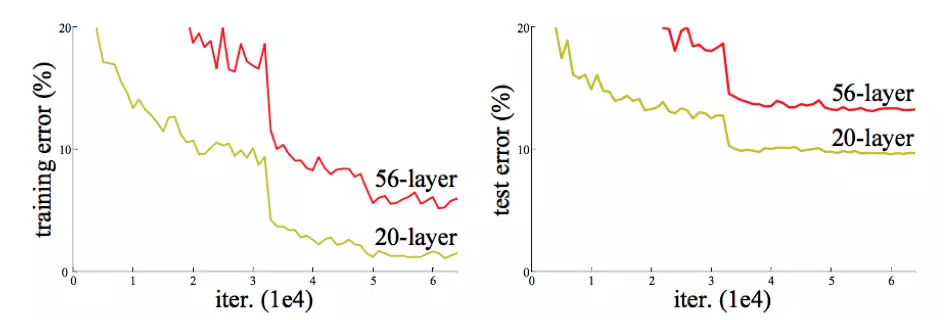深度残差学习（deep residual learning）
对于一个浅层网络，通过向上堆积新层来建立深层网络，一个极端情况是，这些增加的层都作为恒等映射（identity mapping），什么也不学习，仅仅复制浅层网络的特征。在这种情况下，深层网络应该至少和浅层网络性能一样，也不应该出现退化现象。
基于这个假设，作者提出了深度残差学习（deep residual learning）来解决退化问题，残差学习的结构下图所示，类似与电路中的“短路”，恒等链接是一种短路链接（shortcut connection），既不增加额外的参数也不增加计算复杂度。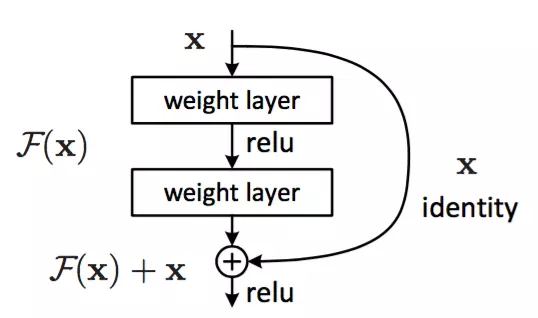对于一个堆积层结构（几层堆积而成），当输入为 $x$ 时其学习到的特征记为 $H(x)$

现在我们希望其可以学习到残差 $F(x)=H(x)-x$ ，相比原始的学习特征 $F(x)+x$，残差学习更容易。

在极端情况下，如果一个恒等映射是最优的，那么将残差置为 0 比使用一堆非线性层来拟合恒等映射更容易，至少网络性能不会下降。

残差不为 0 时，堆积层在输入特征基础上学习到了新的特征，从而拥有更好的性能。

恒等映射块结构
恒等映射（identity mapping）块结构（building block）定义为：
$y = F(x, {W_i}) + x$

$x$ 和 $y$ 是层的输入和输出向量。

函数$F(x, {W_i})$ 表示要学习的残差映射（residual mapping）。

$x$ 和 $F$ 的维度必须是相等的。当更改输入输出通道数不对等时，可以对短路链接执行线性投影$W_s$来匹配维度：
$y = F(x, {W_i }) + W_sx$

对于 ResNet 的两种映射方式，如果网络已经到达最优，继续加深网络，residual mapping 将被 push 为0，只剩下 identity mapping，这样理论上网络一直处于最优状态了，网络的性能也就不会随着深度增加而降低了。

残差函数 $F$ 的形式是可变的，作者提出了两种设计，分别针对 ResNet 34（左图）和 ResNet 50/101/152（右图），一般称整个结构为一个 building block，其中右图又称为 bottleneck design 。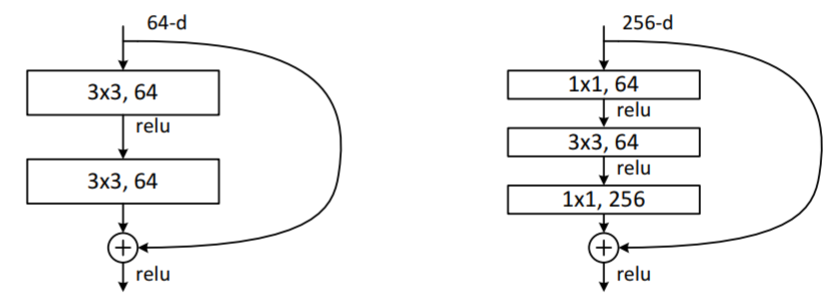Bottleneck Design 通常用于较深的网络中，目的是减少计算和参数量，第一个 $1×1$ 的卷积把 $256$ 维 channel 降到 $64$ 维，最后再通过 $1×1$ 卷积恢复channel 数量。

ResNet 网络架构
查看 resnet-50 网络架构 ：地址
ResNet 网络架构遵循两个简单的设计规则：

特征图输出尺寸相同的层，具有相同数量的滤波器。

如果特征图尺寸减半，则滤波器数量加倍，以便保持每层的时间复杂度。

作者通过步长为 2 的卷积层直接执行下采样。网络以全局平均池化层和具有softmax的1000维全连接层结束。

值得注意的是，ResNet 34与 VGG 网络相比，有更少的滤波器和更低的复杂度。ResNet 34 有 36 亿FLOP(乘加)，仅是VGG-19（196亿FLOP）的18%。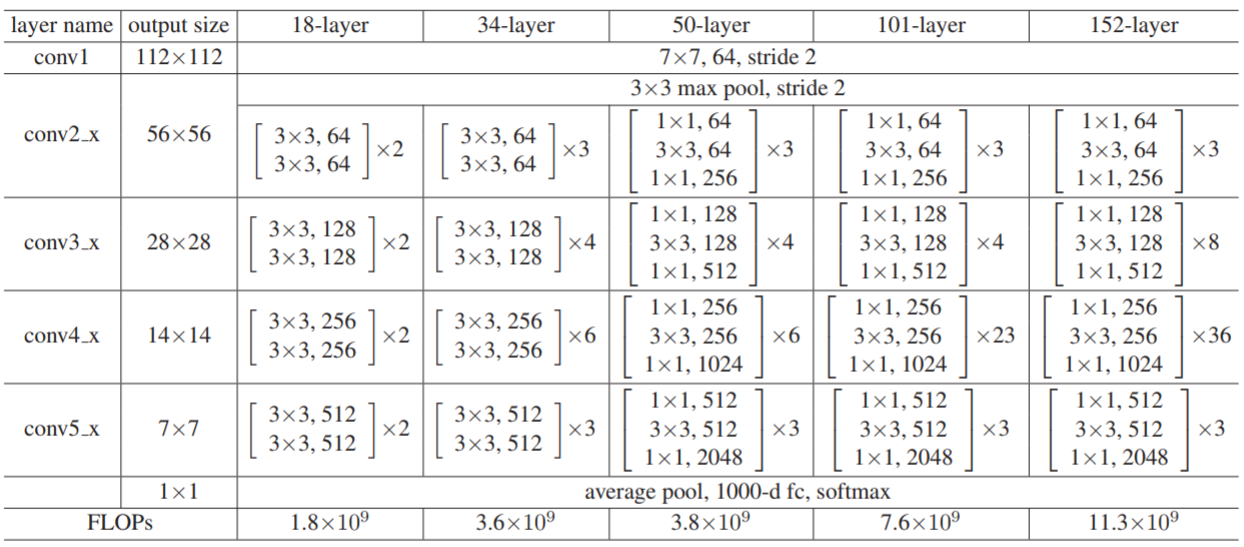作者一共提出了 5 种深度的 ResNet，分别是18、34、50、101和152

所有的网络都分成5部分，分别是：conv1、conv2_x、conv3_x、conv4_x、conv5_x，之后的其他论文也会专门用这个称呼指代 ResNet50 或者 101 的每部分。

以 101-layer 为例，首先经过 $7×7×64$ 的卷积，然后经过$3 + 4 + 23 + 3 = 33$ 个 building block，每个 block 为3层，所以有 $33 × 3 = 99$层，最后是 fc 层(用于分类)，所以一共有 $1 + 99 + 1 = 101$ 层。

注：101层网络仅仅指卷积或者全连接层，而激活层或者Pooling层并没有计算在内；

ResNet 50 微调
ResNet 50 权重文件： 地址
# -*- coding: utf-8 -*-
from keras.optimizers import SGD
from keras.layers import Input, Dense, Convolution2D, MaxPooling2D, AveragePooling2D, ZeroPadding2D, Flatten, Add, Activation
from keras.layers.normalization import BatchNormalization
from keras.models import Model
from keras import backend as K

from sklearn.metrics import log_loss
from get_traffic_dataset import TrafficImageDataGenerator

train_file = './citySpace/outData/train/'
val_file = './citySpace/outData/val/'

def identity_block(input_tensor, kernel_size, filters, stage, block):
"""
The identity_block is the block that has no conv layer at shortcut
Arguments
input_tensor: input tensor
kernel_size: defualt 3, the kernel size of middle conv layer at main path
filters: list of integers, the nb_filters of 3 conv layer at main path
stage: integer, current stage label, used for generating layer names
block: 'a','b'..., current block label, used for generating layer names
"""

nb_filter1, nb_filter2, nb_filter3 = filters
conv_name_base = 'res' + str(stage) + block + '_branch'
bn_name_base = 'bn' + str(stage) + block + '_branch'

x = Convolution2D(nb_filter1, 1, 1, name=conv_name_base + '2a')(input_tensor)
x = BatchNormalization(axis=bn_axis, name=bn_name_base + '2a')(x)
x = Activation('relu')(x)

x = Convolution2D(nb_filter2, kernel_size, kernel_size,
border_mode='same', name=conv_name_base + '2b')(x)
x = BatchNormalization(axis=bn_axis, name=bn_name_base + '2b')(x)
x = Activation('relu')(x)

x = Convolution2D(nb_filter3, 1, 1, name=conv_name_base + '2c')(x)
x = BatchNormalization(axis=bn_axis, name=bn_name_base + '2c')(x)

x = Activation('relu')(x)
return x

def conv_block(input_tensor, kernel_size, filters, stage, block, strides=(2, 2)):
"""
conv_block is the block that has a conv layer at shortcut
# Arguments
input_tensor: input tensor
kernel_size: defualt 3, the kernel size of middle conv layer at main path
filters: list of integers, the nb_filters of 3 conv layer at main path
stage: integer, current stage label, used for generating layer names
block: 'a','b'..., current block label, used for generating layer names
Note that from stage 3, the first conv layer at main path is with subsample=(2,2)
And the shortcut should have subsample=(2,2) as well
"""

nb_filter1, nb_filter2, nb_filter3 = filters
conv_name_base = 'res' + str(stage) + block + '_branch'
bn_name_base = 'bn' + str(stage) + block + '_branch'

x = Convolution2D(nb_filter1, 1, 1, subsample=strides,
name=conv_name_base + '2a')(input_tensor)
x = BatchNormalization(axis=bn_axis, name=bn_name_base + '2a')(x)
x = Activation('relu')(x)

x = Convolution2D(nb_filter2, kernel_size, kernel_size, border_mode='same',
name=conv_name_base + '2b')(x)
x = BatchNormalization(axis=bn_axis, name=bn_name_base + '2b')(x)
x = Activation('relu')(x)

x = Convolution2D(nb_filter3, 1, 1, name=conv_name_base + '2c')(x)
x = BatchNormalization(axis=bn_axis, name=bn_name_base + '2c')(x)

shortcut = Convolution2D(nb_filter3, 1, 1, subsample=strides,
name=conv_name_base + '1')(input_tensor)
shortcut = BatchNormalization(axis=bn_axis, name=bn_name_base + '1')(shortcut)

x = Activation('relu')(x)
return x

def resnet50_model(img_rows, img_cols, color_type=1, num_classes=None):
"""
Resnet 50 Model for Keras
Parameters:
img_rows, img_cols - resolution of inputs
channel - 1 for grayscale, 3 for color
num_classes - number of class labels for our classification task
"""

# Handle Dimension Ordering for different backends
global bn_axis
if K.image_dim_ordering() == 'tf':
bn_axis = 3
img_input = Input(shape=(img_rows, img_cols, color_type))
else:
bn_axis = 1
img_input = Input(shape=(color_type, img_rows, img_cols))

x = Convolution2D(64, 7, 7, subsample=(2, 2), name='conv1')(x)
x = BatchNormalization(axis=bn_axis, name='bn_conv1')(x)
x = Activation('relu')(x)
x = MaxPooling2D((3, 3), strides=(2, 2))(x)

x = conv_block(x, 3, [64, 64, 256], stage=2, block='a', strides=(1, 1))
x = identity_block(x, 3, [64, 64, 256], stage=2, block='b')
x = identity_block(x, 3, [64, 64, 256], stage=2, block='c')

x = conv_block(x, 3, [128, 128, 512], stage=3, block='a')
x = identity_block(x, 3, [128, 128, 512], stage=3, block='b')
x = identity_block(x, 3, [128, 128, 512], stage=3, block='c')
x = identity_block(x, 3, [128, 128, 512], stage=3, block='d')

x = conv_block(x, 3, [256, 256, 1024], stage=4, block='a')
x = identity_block(x, 3, [256, 256, 1024], stage=4, block='b')
x = identity_block(x, 3, [256, 256, 1024], stage=4, block='c')
x = identity_block(x, 3, [256, 256, 1024], stage=4, block='d')
x = identity_block(x, 3, [256, 256, 1024], stage=4, block='e')
x = identity_block(x, 3, [256, 256, 1024], stage=4, block='f')

x = conv_block(x, 3, [512, 512, 2048], stage=5, block='a')
x = identity_block(x, 3, [512, 512, 2048], stage=5, block='b')
x = identity_block(x, 3, [512, 512, 2048], stage=5, block='c')

# Fully Connected Softmax Layer
x_fc = AveragePooling2D((7, 7), name='avg_pool')(x)
x_fc = Flatten()(x_fc)
x_fc = Dense(1000, activation='softmax', name='fc1000')(x_fc)

# Create model
model = Model(img_input, x_fc)

if K.image_dim_ordering() == 'th':
# Use pre-trained weights for Theano backend
weights_path = 'imagenet_models/resnet50_weights_th_dim_ordering_th_kernels.h5'
else:
# Use pre-trained weights for Tensorflow backend
weights_path = 'resnet50_weights_tf_dim_ordering_tf_kernels.h5'

# Truncate and replace softmax layer for transfer learning
# Cannot use model.layers.pop() since model is not of Sequential() type
# The method below works since pre-trained weights are stored in layers but not in the model
x_newfc = AveragePooling2D((7, 7), name='avg_pool')(x)
x_newfc = Flatten()(x_newfc)
x_newfc = Dense(num_classes, activation='softmax', name='fc10')(x_newfc)

# Create another model with our customized softmax
model = Model(img_input, x_newfc)

# Learning rate is changed to 0.001
sgd = SGD(lr=1e-3, decay=1e-6, momentum=0.9, nesterov=True)
model.compile(optimizer=sgd, loss='categorical_crossentropy', metrics=['accuracy'])

return model

if __name__ == '__main__':
# Example to fine-tune on 3000 samples from Cifar10

img_rows, img_cols = 224, 224  # Resolution of inputs
channel = 3
num_classes = 3
batch_size = 16
nb_epoch = 10

# Initalize the data generator seperately for the training and validation set
train_generator = TrafficImageDataGenerator(train_file, horizontal_flip=True, shuffle=True)
val_generator = TrafficImageDataGenerator(val_file, horizontal_flip=True, shuffle=True)

X_valid, Y_valid,val_labels = val_generator.all(10000)
X_train, Y_train, train_labels = train_generator.all(10000)

model = resnet50_model(img_rows, img_cols, channel, num_classes)

# Start Fine-tuning
model.fit(X_train, Y_train,
batch_size=batch_size,
nb_epoch=nb_epoch,
shuffle=True,
verbose=1,
validation_data=(X_valid, Y_valid),
)

# Make predictions
predictions_valid = model.predict(X_valid, batch_size=batch_size, verbose=1)

# Cross-entropy loss score
score = log_loss(Y_valid, predictions_valid)




展开全文• 创建各版本的ResNet模型，ResNet18，ResNet34，ResNet50，ResNet101，ResNet152 原文地址: https://arxiv.org/pdf/1512.03385.pdf 论文就不解读了，大部分解读都是翻译，看的似懂非懂，自己搞懂就行了。最近想着...
创建各版本的ResNet模型，ResNet18，ResNet34，ResNet50，ResNet101，ResNet152
原文地址: https://arxiv.org/pdf/1512.03385.pdf

论文就不解读了，大部分解读都是翻译，看的似懂非懂，自己搞懂就行了。
最近想着实现一下经典的网络结构，看了原文之后，根据原文代码结构开始实现。
起初去搜了下各种版本的实现，发现很多博客都是错误百出，有些博文都发布几年了，错误还是没人发现，评论区几十号人不知道是真懂还是装懂，颇有些无奈啊。
因此打算自己手动实现网络结构，锻炼下自己的代码能力，也加深对网络结构的理解。
写完之后也很欣慰，毕竟一直认为自己是个菜鸡，最近竟然接连不断的发现很多博文的错误之处，而且很多人看后都没发现的，想想自己似乎还有点小水平。
最后在一套代码里，实现了各版本ResNet，为了方便。
其实最后还是觉得应该每个网络分开写比较好。因为不同版本的网络内部操作是有很大差异的，本文下面的代码是将ResidualBlock和			BottleNeckBlock分开写的，但是在维度的变换上差异还是很复杂，一方面想提高代码的复用性，另一方面也受制于复杂度。所以最后写出的算不上高复用性的精简代码。勉强能用。关于ResNet的结构，除各版本分开写之外，重复的block其实也可以分开写，因为BottleNeckBlock的维度变换太复杂，参数变换多，能分开就分开，复杂度小的地方可以复用。

以下是网络结构和实现代码，检验后都是对的；水平有限，如发现有错误，欢迎评论告知！
1 残差结构图2 VGG-19与ResNet34结构比较3 ResNet各版本的结构4 代码实现各版本
import torch.nn as nn
from torch.nn import functional as F

class ResNetModel(nn.Module):
"""
实现通用的ResNet模块，可根据需要定义
"""
def __init__(self, num_classes=1000, layer_num=[],bottleneck = False):
super(ResNetModel, self).__init__()

#conv1
self.pre = nn.Sequential(
#in 224*224*3
#out 112*112*64
nn.BatchNorm2d(64),     #输入通道C = 64

nn.ReLU(inplace=True),   #inplace=True, 进行覆盖操作
# out 112*112*64
# out 56*56*64
)

if bottleneck:  #resnet50以上使用BottleNeckBlock
self.residualBlocks1 = self.add_layers(64, 256, layer_num, 64, bottleneck=bottleneck)
self.residualBlocks2 = self.add_layers(128, 512, layer_num, 256, 2,bottleneck)
self.residualBlocks3 = self.add_layers(256, 1024, layer_num, 512, 2,bottleneck)
self.residualBlocks4 = self.add_layers(512, 2048, layer_num, 1024, 2,bottleneck)

self.fc = nn.Linear(2048, num_classes)
else:   #resnet34使用普通ResidualBlock
self.fc = nn.Linear(512, num_classes)

def add_layers(self, inchannel, outchannel, nums, pre_channel=64, stride=1, bottleneck=False):
layers = []
if bottleneck is False:

#添加大模块首层, 首层需要判断inchannel == outchannel ?
#跨维度需要stride=2，shortcut也需要1*1卷积扩维

layers.append(ResidualBlock(inchannel,outchannel))

#添加剩余nums-1层
for i in range(1,nums):
layers.append(ResidualBlock(outchannel,outchannel))
return nn.Sequential(*layers)
else:   #resnet50使用bottleneck
#传递每个block的shortcut，shortcut可以根据是否传递pre_channel进行推断

#添加首层,首层需要传递上一批blocks的channel
layers.append(BottleNeckBlock(inchannel,outchannel,pre_channel,stride))
for i in range(1,nums): #添加n-1个剩余blocks，正常通道转换，不传递pre_channel
layers.append(BottleNeckBlock(inchannel,outchannel))
return nn.Sequential(*layers)

def forward(self, x):
x = self.pre(x)
x = self.residualBlocks1(x)
x = self.residualBlocks2(x)
x = self.residualBlocks3(x)
x = self.residualBlocks4(x)

x = F.avg_pool2d(x, 7)
x = x.view(x.size(0), -1)
return self.fc(x)

class ResidualBlock(nn.Module):
'''
定义普通残差模块
resnet34为普通残差块，resnet50为瓶颈结构
'''
def __init__(self, inchannel, outchannel, stride=1, padding=1, shortcut=None):
super(ResidualBlock, self).__init__()
#resblock的首层，首层如果跨维度，卷积stride=2，shortcut需要1*1卷积扩维
if inchannel != outchannel:
stride= 2
shortcut=nn.Sequential(
nn.Conv2d(inchannel,outchannel,1,stride,bias=False),
nn.BatchNorm2d(outchannel)
)

# 定义残差块的左部分
self.left = nn.Sequential(
nn.Conv2d(inchannel, outchannel, 3, stride, padding, bias=False),
nn.BatchNorm2d(outchannel),
nn.ReLU(inplace=True),

nn.Conv2d(outchannel, outchannel, 3, 1, padding, bias=False),
nn.BatchNorm2d(outchannel),

)

#定义右部分
self.right = shortcut

def forward(self, x):
out = self.left(x)
residual = x if self.right is None else self.right(x)
out = out + residual
return F.relu(out)

class BottleNeckBlock(nn.Module):
'''
定义resnet50的瓶颈结构
'''
def __init__(self,inchannel,outchannel, pre_channel=None, stride=1,shortcut=None):
super(BottleNeckBlock, self).__init__()
#首个bottleneck需要承接上一批blocks的输出channel
if pre_channel is None:     #为空则表示不是首个bottleneck，
pre_channel = outchannel    #正常通道转换

else:   # 传递了pre_channel,表示为首个block，需要shortcut
shortcut = nn.Sequential(
nn.Conv2d(pre_channel,outchannel,1,stride,0,bias=False),
nn.BatchNorm2d(outchannel)
)

self.left = nn.Sequential(
#1*1,inchannel
nn.Conv2d(pre_channel, inchannel, 1, stride, 0, bias=False),
nn.BatchNorm2d(inchannel),
nn.ReLU(inplace=True),
#3*3,inchannel
nn.Conv2d(inchannel,inchannel,3,1,1,bias=False),
nn.BatchNorm2d(inchannel),
nn.ReLU(inplace=True),
#1*1,outchannel
nn.Conv2d(inchannel,outchannel,1,1,0,bias=False),
nn.BatchNorm2d(outchannel),
nn.ReLU(inplace=True),
)
self.right = shortcut

def forward(self,x):
out = self.left(x)
residual = x if self.right is None else self.right(x)
return F.relu(out+residual)

if __name__ == '__main__':
# channel_nums = [64,128,256,512,1024,2048]

num_classes = 6

#layers = 18, 34, 50, 101, 152
layer_nums = [[2,2,2,2],[3,4,6,3],[3,4,6,3],[3,4,23,3],[3,8,36,3]]
#选择resnet版本，
# resnet18 ——0；resnet34——1,resnet-50——2,resnet-101——3,resnet-152——4
i = 3;
bottleneck = i >= 2   #i<2, false,使用普通的ResidualBlock; i>=2，true,使用BottleNeckBlock

model = ResNetModel(num_classes,layer_nums[i],bottleneck)
print(model)



展开全文pytorch 神经网络 深度学习 python 计算机视觉
• ## ResNetV2：ResNet深度解析

万次阅读 多人点赞 2018-05-09 01:02:01
ResNet残差网络，想必大家一定很熟悉了，那么先考考大家，下面（1）-（5...根据ResNet的描述，似乎以上五组都符合，那么2016年ResNet原文是哪一个结构呢？以及其他四组结构也都work么？我们不禁有了这两个疑问，伴随着
ResNet残差网络，想必大家一定很熟悉了，那么先考考大家，下面（1）-（5）的结构哪个是我们常用的ResNet结构？根据ResNet的描述，似乎以上五组都符合，那么2016年ResNet原文是哪一个结构呢？以及其他四组结构也都work么？我们不禁有了这两个疑问，伴随着疑问我们一一揭开谜题；

图（3）的结构work么？

对于每个图右侧部分我们称作“residual”分支，左侧部分我们称作“identity”分支，如果ReLU作为“residual”分支的结尾，我们不难发现“residual”分支的结果永远非负，这样前向的时候输入会单调递增，从而会影响特征的表达能力，所以我们希望“residual”分支的结果应该在（-∞$\mathrm{\infty }$$\infty$， +∞$\mathrm{\infty }$$\infty$）；这点也是我们以后设计网络时所要注意的。

这里BN改变了“identity”分支的分布，影响了信息的传递，在训练的时候会阻碍loss的下降；这里大家肯定又有个问题：

为什么“identity”分支发生变化，会影响信息传递，从而影响训练呢？

这里简单回顾ResNet的公式：
yl=h(xl)+F(xl,Wl)${y}_{l}=h\left({x}_{l}\right)+F\left({x}_{l},{W}_{l}\right)$$y_l=h(x_l)+F(x_l, W_l)$  and xl+1=f(yl)${x}_{l+1}=f\left({y}_{l}\right)$$x_{l+1} = f(y_l)$
简化以上公式，令所有“identity”分支都是h(xl)=xl$h\left({x}_{l}\right)={x}_{l}$$h(x_l) = x_l$以及xl+1=yl${x}_{l+1}={y}_{l}$$x_{l+1} = y_l$，那么得到:
xl+1=xl+F(xl,Wl)${x}_{l+1}={x}_{l}+F\left({x}_{l},{W}_{l}\right)$$x_{l+1} = x_l + F(x_l, W_l)$
我们递归的计算:
(xl+2=xl+1+F(xl+1,Wl+1)=xl+F(xl,Wl)+F(xl+1,Wl+1)${x}_{l+2}={x}_{l+1}+F\left({x}_{l+1},{W}_{l+1}\right)={x}_{l}+F\left({x}_{l},{W}_{l}\right)+F\left({x}_{l+1},{W}_{l+1}\right)$$x_{l+2} = x_{l+1} + F(x_{l+1}, W_{l+1})=x_l + F(x_l, W_l) + F(x_{l+1}, W_{l+1})$)
xL=xl+∑L−1i=lF(xi,Wi)${x}_{L}={x}_{l}+\sum _{i=l}^{L-1}F\left({x}_{i},{W}_{i}\right)$$x_L = x_l + \sum_{i=l}^{L-1}F(x_i, W_i)$ —— (1)
公式(1)，表达了任何第L层（深层）与第l层（浅层）之间关系；假设损失函数为loss，那么反向传播公式为：
∂loss∂xl=∂loss∂xL∂xL∂xl=∂loss∂xL(1+∂∂xl∑L−1i=lF(xi,Wi))$\frac{\mathrm{\partial }loss}{\mathrm{\partial }{x}_{l}}=\frac{\mathrm{\partial }loss}{\mathrm{\partial }{x}_{L}}\frac{\mathrm{\partial }{x}_{L}}{\mathrm{\partial }{x}_{l}}=\frac{\mathrm{\partial }loss}{\mathrm{\partial }{x}_{L}}\left(1+\frac{\mathrm{\partial }}{\mathrm{\partial }{x}_{l}}\sum _{i=l}^{L-1}F\left({x}_{i},{W}_{i}\right)\right)$$\frac{\partial loss}{\partial x_l} = \frac{\partial loss}{\partial x_L} \frac{\partial x_L}{\partial x_l} = \frac{\partial loss}{\partial x_L} (1 + \frac{\partial}{\partial x_l}\sum_{i=l}^{L-1}F(x_i, W_i))$
这个反向传播公式有几个特点：（1）关于xl${x}_{l}$$x_l$的梯度信息与两部分值有关：xL${x}_{L}$$x_L$的梯度值，也就是说两层之间梯度信息无障碍传递了，以及∂loss∂xL∂∂xl∑L−1i=lF(xi,Wi))$\frac{\mathrm{\partial }loss}{\mathrm{\partial }{x}_{L}}\frac{\mathrm{\partial }}{\mathrm{\partial }{x}_{l}}\sum _{i=l}^{L-1}F\left({x}_{i},{W}_{i}\right)\right)$$\frac{\partial loss}{\partial x_L} \frac{\partial}{\partial x_l}\sum_{i=l}^{L-1}F(x_i, W_i))$; （2）∂loss∂xl$\frac{\mathrm{\partial }loss}{\mathrm{\partial }{x}_{l}}$$\frac{\partial loss}{\partial x_l}$的值不会轻易抵消因为在一个mini-batch中，∂∂xl∑L−1i=lF(xi,Wi))$\frac{\mathrm{\partial }}{\mathrm{\partial }{x}_{l}}\sum _{i=l}^{L-1}F\left({x}_{i},{W}_{i}\right)\right)$$\frac{\partial}{\partial x_l}\sum_{i=l}^{L-1}F(x_i, W_i))$不会一直为-1；（3）有效的防止了当权重很小时，梯度消失的问题。
以上优秀的特点只有在假设h(xl)=xl$h\left({x}_{l}\right)={x}_{l}$$h(x_l) = x_l$以及xl+1=yl${x}_{l+1}={y}_{l}$$x_{l+1} = y_l$成立时才有效，所以ResNet要尽量保证两点：1）不轻易改变”identity“分支的值，也就是输入与输出一致；2）addition之后不再接改变信息分布的层；
到此也就彻底回答了图（2）的结构为何会阻碍反向传播时的信息。

在分析图（4）和图（5）之前，我们引出一个概念：”Post-activation”和”Pre-activation”，其中Post和Pre的概念是相对于weight(conv)层来说的，那么我们不难发现，图(1), (2), (3)都是”Post-activation”，图(4), (5)都是”Pre-activation”，那么两种模式哪一个更好呢？这里我们就用实验结果说话。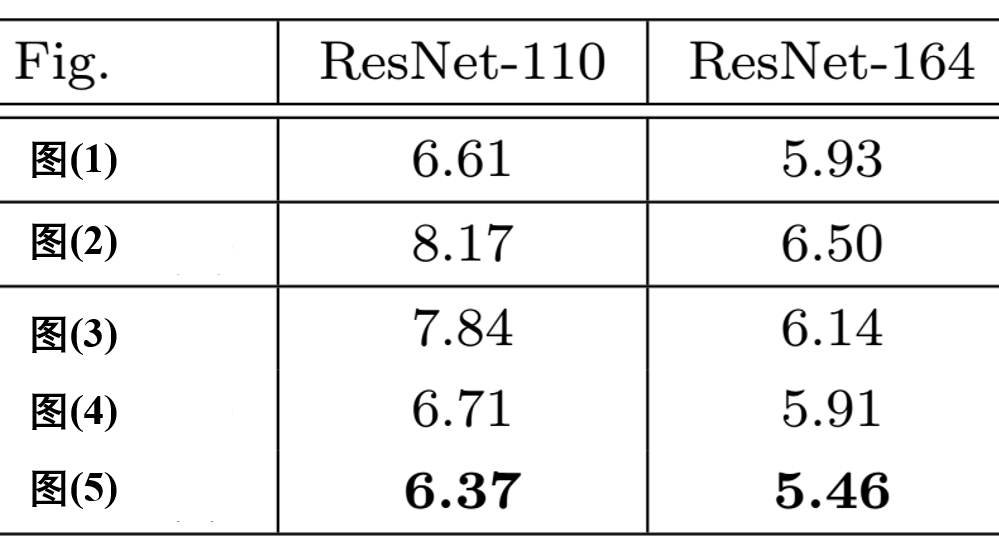上图是5种结构在Cifar10上的实验结果，一共实验了两种网络ResNet110和ResNet164（注：这里直接摘抄了原文的图片，本人并没有真实的试验过）；
从实验结果上，我们可以发现图(4)的结构与ResNet原结构伯仲之间，稍稍逊色，然而图(5)的结构却好于ResNet原结构。图5的结构好的原因在于两点：1）反向传播基本符合假设，信息传递无阻碍；2）BN层作为pre-activation，起到了正则化的作用；

最后我们通常把图5的结构称作ResNetV2，这里我们把ResNetV1和ResNetV2结构再次show：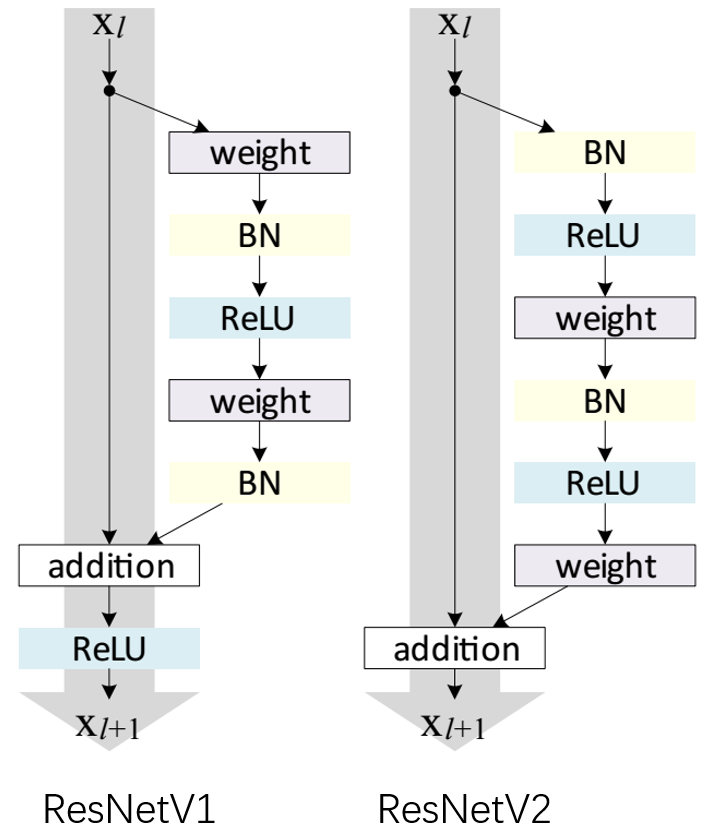展开全文深度学习与计算机视觉
• 在FPN 原文Feature Pyramid Networks for Object Detection中，使用了ResNet原文 Deep Residual Learning for Image Recognition中的resnet34层模型的 conv2到conv5，conv2到conv5的的维度分别是64，128，256，512。...
• 首先附上ResNet原文地址Deep Residual Learning for Image Recognition 其中，ResNet整体网络结构图如下： 一、ResNet网络中的两种基本残差块 由网络结构图可以看出，ResNet-18和ResNet-3...
• 原文链接：https://blog.csdn.net/u013181595/article/details/80990930 ResNet（Residual Neural Network）由微软研究院的Kaiming He等四名华人提出，通过使用ResNet Unit成功训练出了152层的神经网络，并在ILSVRC...神经网络 网络 卷积 深度学习
• ResNet 原文：https://arxiv.org/pdf/1512.03385.pdf 摘要 更深的神经网络更难训练。 我们提出了一个残差学习框架，以减轻网络训练的负担，这些网络比以前使用的网络要深得多。我们明确地将这些层重新定义为根据...
• 这篇教程我们来实现Kaiming He大神提出的ResNet...ResNet原文： Deep Residual Learning for Image Recognition 这篇文章中提出了像下面这样的经典残差结构，关于这种结构的解读可搜索其他文章，在此不多赘述。 ...tensorflow
• ResNet原文在这里。2015年，微软亚洲研究院提出ResNet网络，以3.75%的top-5的错误率获得当时的ILSCRC大赛冠军。（值得一提的是ResNet的提出者何凯明大神是清华毕业的，2003年广东省理科状元，绝对的是中国人在AI圈的...
• Deep Residual Learning for Image Recognition--ResNet 原文  点击打开链接 “ease the training of networks that are substantially deeper than those used previously” 核心思想：提高更深层网络的训练...
• 阅读ResNet论文原文 搜集学习资源：视频讲解-博客资源 熟悉ResNet网络结构 代码复现，清楚网络结构中层与层之间的操作 ResNet论文 原论文：2015-Deep Residual Learning for Image Recognition 2016-Identity ...深度学习 神经网络 tensorflow
• 原文地址： resnet文章 传统思路 在resnet还没有被提出来的时候，通常的思路是通过堆叠更多的层就能实现。但是随着网络深度的增加，就会发现不仅网络模型会变得非常大，而且会暴露出一个问题，即退化问题：随着网络...
• [论文笔记] ResNet:Deep Residual Learning for Image Recognition说在前面个人心得： 1. 学习残差比学习一个未知的函数要容易 2.... 在复现过程中，发现ResNet的没有biasCVPR 2016，原文链接：https:...
• 作者：binlearning 原文地址：...ResNet-v2 Identity Mappings in Deep Residual NetworksKaiming He, Xiangyu Zhang, Shaoqing Ren, Jian Sun Caffe实现：https://github.com/binLea...
• ## PyTorch ResNet 测试

千次阅读 2019-04-26 18:21:00
原文： https://blog.csdn.net/shanglianlm/article/details/86376627 resnet50 1070 gpu上需要13-15ms resnet18p100 512*512 gpu上需要6ms resnet34: p100 512*512 gpu 10ms 1070 1 416*416 gpu 11ms 1070 8...
• 在计算机视觉里，特征的“等级”随增网络深度的加深而变高，研究表明，网络的深度是实现好的效果的重要因素。然而梯度弥散/爆炸成为训练深层次的网络的障碍，导致无法收敛。...原文链接：https://blog.csdn.net/mao_...
• 原文链接：点击打开链接摘要： ResNet论文笔记 一、论文简介先从Kaiming He的那篇paper说起吧，paper主要解决的还是神经网络的深度和准确率之间的关系，当神经网络的层数增加，出现了degradation，trainging error...
• 一.Resnet网络架构 1.plain network （原文表述）Plain Network. Our plain baselines (Fig. 3, middle) aremainly inspired by the philosophy of VGG nets  (Fig. 3,left). The convolutional layers mostly...
• 3 x 3 resnet_block[ 64 ] x2 identity x 2 3 x 3 resnet_block[ 128 ] x2 identity x 2 3 x 3 resnet_block[ 256 ] x2 identity x 2 3 x 3 resnet_block[ 512 ] x2 identity x 2 avgpool fc ——————————...
• 原文地址：...结构有ResNet 50、ResNet 152、ResNet 200，考虑耗时原因只跑了ResNet 152网络结构的forward。 # coding:UTF-8 """ Typical use: from tensorflow.contrib.sli...
• 本文系转载，感谢原文作者的分享。 【深度学习】入门理解ResNet和他的小姨子们（一）—ResNet http://blog.csdn.net/shwan_ma/article/details/78163921【深度学习】入门理解ResNet和他的小姨子们（二）—DenseNet ...深度学习
• 目录 1. ResNet解决了什么问题 2. ResNet原理及结构 ...博客中的ResNet内容来自何凯明大神在CVPR2016发表的文章《Deep Residual Learning for Image Recognition》（原文链接），ResNet代码部分来自Pytopytorch 深度学习
• ## ResNet论文详解

万次阅读 2018-11-19 20:28:53
原文地址： https://arxiv.org/pdf/1512.03385.pdf 论文目标： 提出一种方法使更深的卷积神经网络的训练成为可能。 论文工作： 将网络层要学习的函数关系变为学习关于层输入的残差函数，不是学习没有参考的...深度学习 计算机视觉 图像处理
• 原文链接：残差网络ResNet笔记 1. 思想 作者根据输入将层表示为学习残差函数。实验表明，残差网络更容易优化，并且能够通过增加相当的深度来提高准确率。 核心是解决了增加深度带来的副作用（退化问题），这样能够...深度学习 残差网络
• 目录 1.resnet 简述 2.网络结构 3.训练模型 1.resnet 简述 ...Resnet是残差网络(Residual Network)的缩写,该...原文链接：https://arxiv.org/abs/1512.03385 2.网络结构 网络结构如图，resnet50分为conv1、con.卷积 神经网络 pytorch 深度学习...